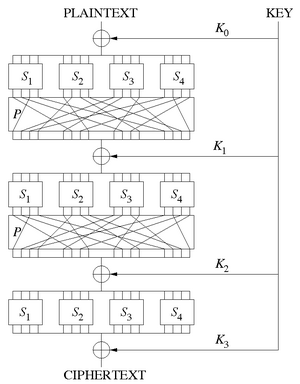# Substitution-permutation network facts for kids

Kids Encyclopedia FactsA sketch of a Substitution-Permutation Network with 3 rounds, encrypting a plaintext block of 16 bits into a ciphertext block of 16 bits. The S-boxes are the Si’s, the P-boxes are the same P, and the round keys are the Ki’s.

In cryptography, an SP-network, or substitution-permutation network (SPN), is a series of linked mathematical operations used in block cipher algorithms such as AES.

These networks consist of S-boxes and P-boxes that transform blocks of input bits into output bits.

S-boxes substitute or transform input bits into output bits. A good S-box will have the property that changing one input bit will change about half of the output bits. It will also have the property that each output bit will depend on every input bit. P-boxes permute or rearrange bits across S-box inputs. In addition, at each round the key is combined using some group operation, typically XOR.

## Other pagesSubstitution-permutation network Facts for Kids. Kiddle Encyclopedia.